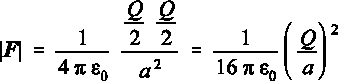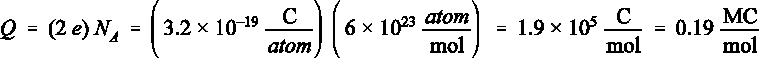# Physics 1028 Chapter Notes - Chapter 17: Mechanical Equilibrium, Electric Field

2 views5 pages
School
Western University
Department
Physics
Course
Physics 1028
ProfessorSelection of Even–Numbered Problem Solutions
Chapter 17
P–17.2
Initially, there is no electrostatic force since sphere B doesn’t carry a charge. After connecting both spheres,
the mutual repulsion of the mobile positive charges on sphere A leads to a maximum charge separation, given
when qA = Q/2 and qB = Q/2.
At this point we make a less obvious assumption: We assume that the uniformity of the charge dis-
tribution on either sphere is not disturbed by the other sphere. This assumption is reasonably justified as long
as the sizes of the two spheres are small in comparison to their distance. When this assumption is valid, the
entire charge on either sphere behaves as if it were a point charge at the centre of the sphere. Note that we
would have to consider all the individual point charges on each sphere separately without this assumption.
Using Coulomb’s law we find for the magnitude of the force between the two spheres:
(1)
This is a repulsive force because both spheres are positive.
Unlock document

This preview shows pages 1-2 of the document.
Unlock all 5 pages and 3 million more documents.P–17.4
The electrons in the atomic shell of helium are electrically balanced by the two protons in the nucleus. Thus,
the total amount of negative charge is:
(2)
Note that there are also two neutrons in each helium nucleus. Free neutrons decay into an electron and a
proton with a half–life of 14 minutes. In the above calculation we neglected these additional electrons because
neutrons in a nucleus are stable.
Unlock document

This preview shows pages 1-2 of the document.
Unlock all 5 pages and 3 million more documents.

## Document Summary

Initially, there is no electrostatic force since sphere b doesn"t carry a charge. After connecting both spheres, the mutual repulsion of the mobile positive charges on sphere a leads to a maximum charge separation, given when qa = q/2 and qb = q/2. At this point we make a less obvious assumption: we assume that the uniformity of the charge dis- tribution on either sphere is not disturbed by the other sphere. This assumption is reasonably justified as long as the sizes of the two spheres are small in comparison to their distance. When this assumption is valid, the entire charge on either sphere behaves as if it were a point charge at the centre of the sphere. Note that we would have to consider all the individual point charges on each sphere separately without this assumption. Using coulomb"s law we find for the magnitude of the force between the two spheres: (1)